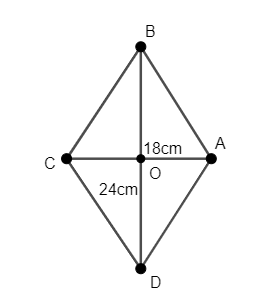QuestionAnswers

# The length of the diagonals of a rhombus is 24cm and 18cm. Find the length of each side of the rhombus.Hint:Calculate the half-length of both the diagonals of the rhombus. Use the fact that the diagonals of a rhombus bisect each other and then use Pythagoras Theorem to calculate the length of each side of the rhombus.

We have to calculate the length of each side of a rhombus whose diagonals are of length 18cm and 24cm.
We will consider the rhombus ABCD whose diagonals intersect at point O such that $BD=24cm$ and $AC=18cm$, as shown in the figure.We will first calculate the half-length of both the diagonals of the rhombus.
We know that the diagonals of a rhombus bisect each other.
Thus, we have $OB=OD=\dfrac{24}{2}=12cm$ and $OA=OC=\dfrac{18}{2}=9cm$.
As the diagonals bisect each other, we have $OA\bot OB$. Thus, $\Delta OAB$ is a right-angled triangle.
We will now use Pythagoras Theorem to calculate the length of each side of the rhombus.
We know that Pythagoras Theorem states that in a right-angled triangle, the sum of squares of two perpendicular sides is equal to the square of the third side.
Thus, in $\Delta OAB$, we have ${{\left( OA \right)}^{2}}+{{\left( OB \right)}^{2}}={{\left( AB \right)}^{2}}$.
Substituting $OA=9cm,OB=12cm$ in the above formula, we have ${{\left( 12 \right)}^{2}}+{{9}^{2}}=A{{B}^{2}}$.
Thus, we have $A{{B}^{2}}={{12}^{2}}+{{9}^{2}}=144+81=225$.
Taking square root on both sides, we have $AB=\sqrt{225}=15cm$.
We know that the length of all sides of a rhombus is equal. Thus, we have $AB=BC=CD=AD=15cm$.
Hence, the length of each side of the rhombus is 15cm.

Note: We can calculate the length of each side of rhombus by applying Pythagoras Theorem in any of the right-angled triangles. We will get the same answer in each case. We don’t have to calculate the length of each side of the rhombus. We can simply use the fact that the length of all sides of a rhombus is equal.Remember that the length of diagonals of rhombus are always different and they only bisect with each other.
View Notes
Area of a Rhombus FormulaRelation Between the Length of a Given Wire and Tension for Constant Frequency Using SonometerWhat is the full form of phd?The Making of a ScientistChanging the Period of a PendulumThe Difference Between an Animal that is A Regulator and One that is A ConformerThe Idea of TimeMeasurement of Length DistanceMeasuring the Rate of Change of MotionThe Perimeter of Rectangle FormulaImportant Questions for CBSE Class 7 English Honeycomb Chapter 10 - The Story of CricketImportant Questions for CBSE Class 8 Science Chapter 10 - Reaching The Age of AdolescenceCBSE Class 8 Science Reaching The Age of Adolescence WorksheetsImportant Questions for CBSE Class 6 English A Pact with The Sun Chapter 6 - The Monkey and the CrocodileImportant Questions for CBSE Class 6 English A Pact with The Sun Chapter 1 - A Tale of Two BirdsImportant Questions for CBSE Class 6 English A Pact with The Sun Chapter 8 - A Pact with the SunImportant Questions for CBSE Class 6 English A Pact with The Sun Chapter 10 - A Strange Wrestling MatchImportant Questions for CBSE Class 6 Social Science The Earth Our Habitat Chapter 3 - Motions of the EarthImportant Questions for CBSE Class 6 English A Pact with The Sun Chapter 2 - The Friendly MongooseImportant Questions for CBSE Class 11 English Snapshots Chapter 1 - The Summer of the Beautiful White HorseCBSE Class 10 Hindi A Question Paper 2020Hindi A Class 10 CBSE Question Paper 2009Hindi A Class 10 CBSE Question Paper 2015Previous Year Question Paper of CBSE Class 10 EnglishHindi A Class 10 CBSE Question Paper 2016Hindi A Class 10 CBSE Question Paper 2012Hindi A Class 10 CBSE Question Paper 2010Hindi A Class 10 CBSE Question Paper 2008Hindi A Class 10 CBSE Question Paper 2014Hindi A Class 10 CBSE Question Paper 2007NCERT Solutions for Class 10 Social Science India and the Contemporary World - II Chapter 4 - The Making of a Global WorldNCERT Solutions for Class 10 English Footprints Without Feet Chapter 6 - The Making of a ScientistNCERT Solutions for Class 10 Social Science India and the Contemporary World - II Chapter 5 - The Age of IndustrialisationRS Aggarwal Class 11 Solutions Chapter-16 Conditional Identities Involving the Angles of a TriangleNCERT Solutions for Class 10 Social Science India and the Contemporary World - II Chapter 1 - The Rise of Nationalism in EuropeNCERT Solutions for Class 9 English Beehive Chapter 5 - A Legend of The NorthlandNCERT Solutions for Class 6 English A pact with the Sun Chapter-10NCERT Exemplar for Class 8 Science Solutions Chapter 10 Reaching the Age of AdolescenceNCERT Solutions Class 11 English Woven Words Poem Chapter 10 Felling of the Banyan TreeNCERT Solutions Class 11 English Woven Words Prose Chapter 4 The Adventure of the Three Garridebs